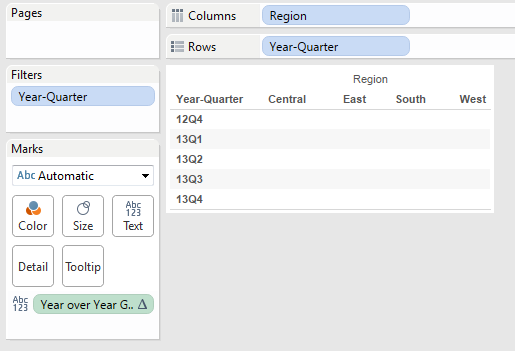# Tableau - Real Time Scenario 8 (How to calculate Year over Year Growth when quarters are like 15Q1, 15Q2...)

Problem: How to calculate Year over Year Growth when quarters are like 15Q1, 15Q2......

For problem description see below imageSolution:

Note: I don’t have Quarter like 15Q1, 15Q2 ….. in sample super store data source. So I am creating calculation field as “Year-Quarter” using below formula.

right(str(year([Order Date])),2) + 'Q' + str(datepart('quarter',[Order Date]))

Step 1: User want to see current quarter and last 4 previous quarter. So darg “Year-Quarter” column to filter shelf and select last 5 quarters in the filter list. I.e 12Q4, 13Q1, 13Q2, 13Q3 and 13Q4.

Step 2: Now create a calculate field “Year over Year Growth” using below formula.

(sum(Sales) – lookup(Sum(Sales),-4))/lookup(Sum(Sales),-4)

See explanation below:Step 3: Drag Year-Quarter Column to Row Shelf and Region Column to Column Shelf.

Step 4: Drag “Year over Year Growth” field to Text Shelf
see belowIn above image you are seeing blanks for each each Quarter. Because by default Year over Year Growthtable calculation computing across table. We need to compute this along Table Down. See belowThen you will see like belowStep 5: Right click on Year over Year Growth field which is there in text shelf and change number format to percentage.

Note: User wants to see only last row. Because Other rows showing blank.

Step 6: Create a Calculate filed “Show Last Row” using below formula.

if last() = 0 then "Show" else "Hide" END

Note: In above formula, some people may directly use “last() = 0” only instead of entire formula which I used. I like this kind of usage in formula. In future maintenance people can understand easily, that we are doing some show and hide functionality.

Step 7: Drag “Show Last Row” field to filter shelf and select “Show”. After applying filter you will see like belowThis is not desired output. “Show Last Row” is computing along table acrossRight click on “Show Last Row” which is there in filter shelf, go to compute using and selectTable Down then it will pop up once again for filter selection. Select “Show”. Click OKbutton.

Here you will see final output: